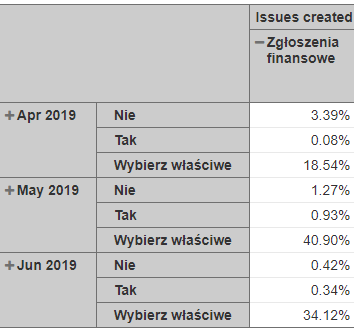# Add calculated - % of total

Hi!
After update finally we got % of total calculation.
I would like to ask how to edit function to count total as one month, not as total of all months:
Like in this case - i want to count % of total issues per month not per total:I guess i need to change standard calculation, but need your help where and what to write ? MDX is still a mystery for meHi,

In your case, try the following code:

``````CASE WHEN [Measures].[Issues created] = 0 THEN 0
WHEN NOT IsEmpty([Measures].[Issues created]) THEN
[Measures].[Issues created]/
(
[Dimension2].DefaultMember, --dimension used in rows (after Time)
[Measures].[Issues created]
)
END
``````

Since I don’t see the name of your dimensions in rows (only values are visible in the screenshot: Nie, Tak, etc) I am using the Dimension2 in my code example, but you should use the dimension that you use in rows (after “Time”)

Martins / eazyBI support

1 Like

Perfect! Thank you!